# Passive Filter Circuits

## AC Electric Circuits

• #### Question 1

In very simple, qualitative terms, rate the impedance of capacitors and inductors as “seen” by low-frequency and high-frequency signals alike:

Capacitor as it “appears” to a low frequency signal: (high or low) impedance?
Capacitor as it “appears” to a high frequency signal: (high or low) impedance?
Inductor as it “appears” to a low frequency signal: (high or low) impedance?
Inductor as it “appears” to a high frequency signal: (high or low) impedance?
• #### Question 2

Identify these filters as either being “low-pass” or “high-pass”, and be prepared to explain your answers: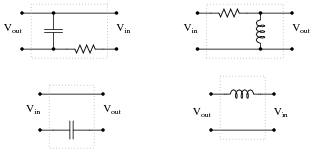• #### Question 3

Suppose you were installing a high-power stereo system in your car, and you wanted to build a simple filter for the “tweeter” (high-frequency) speakers so that no bass (low-frequency) power is wasted in these speakers. Modify the schematic diagram below with a filter circuit of your choice: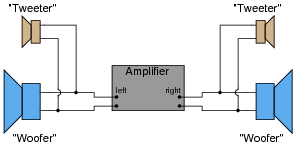Hint: this only requires a single component per tweeter!

• #### Question 4

It is common in audio systems to connect a capacitor in series with each “tweeter” (high-frequency) speaker to act as a simple high-pass filter. The choice of capacitors for this task is important in a high-power audio system.

A friend of mine once had such an arrangement for the tweeter speakers in his car. Unfortunately, though, the capacitors kept blowing up when he operated the stereo at full volume! Tired of replacing these non-polarized electrolytic capacitors, he came to me for advice. I suggested he use mylar or polystyrene capacitors instead of electrolytics. These were a bit more expensive than electrolytic capacitors, but they did not blow up. Explain why.

• #### Question 5

Suppose a friend wanted to install filter networks in the “woofer” section of their stereo system, to prevent high-frequency power from being wasted in speakers incapable of reproducing those frequencies. To this end, your friend installs the following resistor-capacitor networks: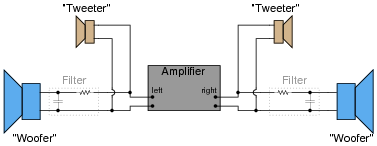After examining this schematic, you see that your friend has the right idea in mind, but implemented it incorrectly. These filter circuits would indeed block high-frequency signals from getting to the woofers, but they would not actually accomplish the stated goal of minimizing wasted power.

What would you recommend to your friend in lieu of this circuit design?

• #### Question 6

The superposition principle describes how AC signals of different frequencies may be “mixed” together and later separated in a linear network, without one signal distorting another. DC may also be similarly mixed with AC, with the same results.

This phenomenon is frequently exploited in computer networks, where DC power and AC data signals (on-and-off pulses of voltage representing 1-and-0 binary bits) may be combined on the same pair of wires, and later separated by filter circuits, so that the DC power goes to energize a circuit, and the AC signals go to another circuit where they are interpreted as digital data:Filter circuits are also necessary on the transmission end of the cable, to prevent the AC signals from being shunted by the DC power supply’s capacitors, and to prevent the DC voltage from damaging the sensitive circuitry generating the AC voltage pulses.

Draw some filter circuits on each end of this two-wire cable that perform these tasks, of separating the two sources from each other, and also separating the two signals (DC and AC) from each other at the receiving end so they may be directed to different loads: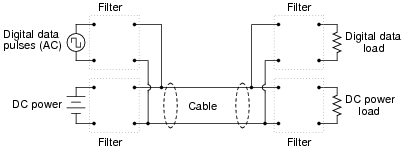• #### Question 7

The following schematic shows the workings of a simple AM radio receiver, with transistor amplifier:The “tank circuit” formed of a parallel-connected inductor and capacitor network performs a very important filtering function in this circuit. Describe what this filtering function is.

• #### Question 8

Draw the Bode plot for an ideal high-pass filter circuit:Be sure to note the “cutoff frequency” on your plot.

• #### Question 9

Draw the Bode plot for an ideal low-pass filter circuit: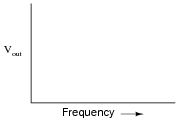Be sure to note the “cutoff frequency” on your plot.

• #### Question 10

Identify what type of filter this circuit is, and calculate its cutoff frequency given a resistor value of 1 kΩ and a capacitor value of 0.22 μF: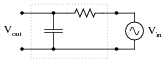Calculate the impedance of both the resistor and the capacitor at this frequency. What do you notice about these two impedance values?

• #### Question 11

Identify what type of filter this circuit is, and calculate its cutoff frequency: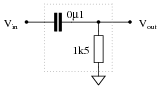• #### Question 12

Identify what type of filter this circuit is, and calculate its cutoff frequency: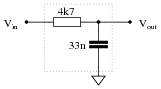• #### Question 13

Identify what type of filter this circuit is, calculate its cutoff frequency, and distinguish the input terminal from the output terminal: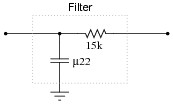• #### Question 14

The formula for determining the cutoff frequency of a simple LR filter circuit looks substantially different from the formula used to determine cutoff frequency in a simple RC filter circuit. Students new to this subject often resort to memorization to distinguish one formula from the other, but there is a better way.

In simple filter circuits (comprised of one reactive component and one resistor), cutoff frequency is that frequency where circuit reactance equals circuit resistance. Use this simple definition of cutoff frequency to derive both the RC and the LR filter circuit cutoff formulae, where fcutoff is defined in terms of R and either L or C.

• #### Question 15

Identify what type of filter this circuit is, and calculate the size of resistor necessary to give it a cutoff frequency of 3 kHz:• #### Question 16

Calculate the power dissipated by this circuit’s load at two different source frequencies: 0 Hz (DC), and fcutoff.What do these figures tell you about the nature of this filter circuit (whether it is a low-pass or a high-pass filter), and also about the definition of cutoff frequency (also referred to as f−3 dB)?

• #### Question 17

Filter circuits don’t just attenuate signals, they also shift the phase of signals. Calculate the amount of phase shift that these two filter circuits impart to their signals (from input to output) operating at the cutoff frequency: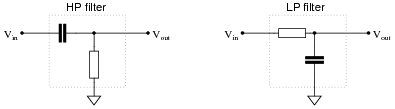• #### Question 18

Real filters never exhibit perfect “square-edge” Bode plot responses. A typical low-pass filter circuit, for example, might have a frequency response that looks like this: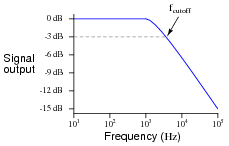What does the term rolloff refer to, in the context of filter circuits and Bode plots? Why would this parameter be important to a technician or engineer?

• #### Question 19

Explain what a band-pass filter is, and how it differs from either a low-pass or a high-pass filter circuit. Also, explain what a band-stop filter is, and draw Bode plots representative of both band-pass and band-stop filter types.

• #### Question 20

A common way of representing complex electronic systems is the block diagram, where specific functional sections of a system are outlined as squares or rectangles, each with a certain purpose and each having input(s) and output(s). For an example, here is a block diagram of an analog (“Cathode Ray”) oscilloscope, or CRO: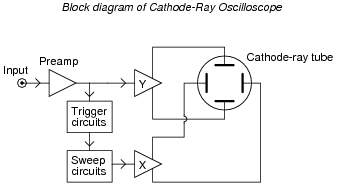Block diagrams may also be helpful in representing and understanding filter circuits. Consider these symbols, for instance: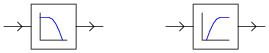Which of these represents a low-pass filter, and which represents a high-pass filter? Explain your reasoning.

Also, identify the new filter functions created by the compounding of low- and high-pass filter “blocks”: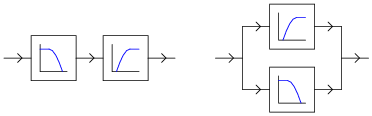• #### Question 21

What kind of filtering action (high-pass, low-pass, band-pass, band-stop) does this resonant circuit provide?• #### Question 22

What kind of filtering action (high-pass, low-pass, band-pass, band-stop) does this resonant circuit provide?• #### Question 23

Identify each of these filter types, and explain how you were able to positively identify their behaviors: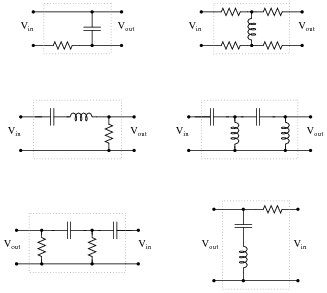• #### Question 24

Identify the following filter types, and be prepared to explain your answers: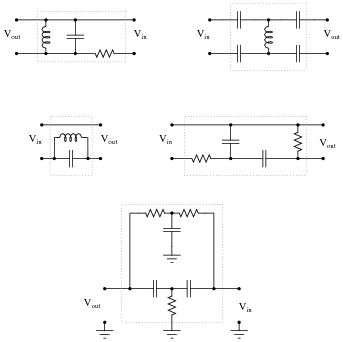• #### Question 25

The cutoff frequency, also known as half-power point or -3dB point, of either a low-pass or a high-pass filter is fairly easy to define. But what about band-pass and band-stop filter circuits? Does the concept of a “cutoff frequency” apply to these filter types? Explain your answer.

• #### Question 26

Plot the typical response of a band-pass filter circuit, showing signal output (amplitude) on the vertical axis and frequency on the horizontal axis:Also, identify and label the bandwidth of the circuit on your filter plot.

• #### Question 27

Plot the typical response of a band-stop filter circuit, showing signal output (amplitude) on the vertical axis and frequency on the horizontal axis:Also, identify and label the bandwidth of the circuit on your filter plot.

• #### Question 28

Plot the typical frequency responses of four different filter circuits, showing signal output (amplitude) on the vertical axis and frequency on the horizontal axis:Also, identify and label the bandwidth of the filter circuit on each plot.

• #### Question 29

A white noise source is a special type of AC signal voltage source which outputs a broad band of frequencies (“noise”) with a constant amplitude across its rated range. Determine what the display of a spectrum analyzer would show if directly connected to a white noise source, and also if connected to a low-pass filter which is in turn connected to a white noise source: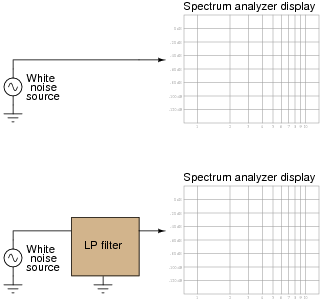• #### Question 30

Predict how the operation of this second-order passive filter circuit will be affected as a result of the following faults. Consider each fault independently (i.e. one at a time, no multiple faults):Capacitor C1 fails open:
Capacitor C2 fails shorted:
Resistor R1 fails open:
Resistor R2 fails open:
Solder bridge (short) across resistor R2:

For each of these conditions, explain why the resulting effects will occur.

• #### Question 31

The Q factor of a series inductive circuit is given by the following equation:

 Q = XL Rseries

Likewise, we know that inductive reactance may be found by the following equation:

 XL = 2 πf L

We also know that the resonant frequency of a series LC circuit is given by this equation:

fr = 1

 2 π √ LC

Through algebraic substitution, write an equation that gives the Q factor of a series resonant LC circuit exclusively in terms of L, C, and R, without reference to reactance (X) or frequency (f).

• #### Question 32

Calculate the resonant frequency, bandwidth, and half-power points of the following filter circuit:• #### Question 33

Suppose a few turns of wire within the inductor in this filter circuit suddenly became short-circuited, so that the inductor effectively has fewer turns of wire than it did before: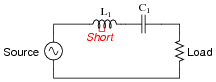What sort of effect would this fault have on the filtering action of this circuit?

• #### Question 34

An interesting technology dating back at least as far as the 1940’s, but which is still of interest today is power line carrier: the ability to communicate information as well as electrical power over power line conductors. Hard-wired electronic data communication consists of high-frequency, low voltage AC signals, while electrical power is low-frequency, high-voltage AC. For rather obvious reasons, it is important to be able to separate these two types of AC voltage quantities from entering the wrong equipment (especially the high-voltage AC power from reaching sensitive electronic communications circuitry).

Here is a simplified diagram of a power-line carrier system: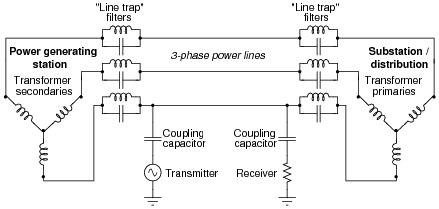The communications transmitter is shown in simplified form as an AC voltage source, while the receiver is shown as a resistor. Though each of these components is much more complex than what is suggested by these symbols, the purpose here is to show the transmitter as a source of high-frequency AC, and the receiver as a load of high-frequency AC.

Trace the complete circuit for the high-frequency AC signal generated by the “Transmitter” in the diagram. How many power line conductors are being used in this communications circuit? Explain how the combination of “line trap” LC networks and “coupling” capacitors ensure the communications equipment never becomes exposed to high-voltage electrical power carried by the power lines, and visa-versa.

• #### Question 35

In this power-line carrier system, a pair of coupling capacitors connects a high-frequency “Transmitter” unit to two power line conductors, and a similar pair of coupling capacitors connects a “Receiver” unit to the same two conductors: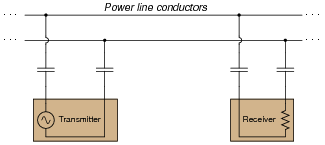While coupling capacitors alone are adequate to perform the necessary filtering function needed by the communications equipment (to prevent damaged from the high-voltage electrical power also carried by the lines), that signal coupling may be made more efficient by the introduction of two line tuning units: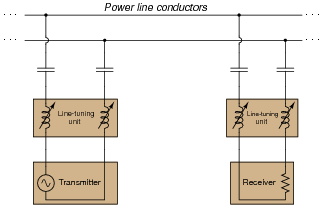Explain why the addition of more components (in series, no less!) provides a better “connection” between the high-frequency Transmitter and Receiver units than coupling capacitors alone. Hint: the operating frequency of the communications equipment is fixed, or at least variable only over a narrow range.

• #### Question 36

The following circuit is called a twin-tee filter:Research the equation predicting this circuit’s “notch” frequency, given the component value ratios shown.

 fnotch =

• #### Question 37

Suppose this band-stop filter were to suddenly start acting as a high-pass filter. Identify a single component failure that could cause this problem to occur: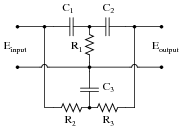• #### Question 38

Examine the following schematic diagram for an audio tone control circuit:Determine which potentiometer controls the bass (low frequency) tones and which controls the treble (high frequency) tones, and explain how you made those determinations.

• #### Question 39

Examine the following audio tone control circuit, used to control the balance of bass and treble heard at the headphones from an audio source such as a radio or CD player: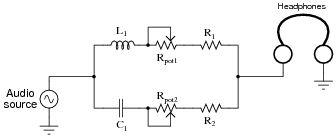Suppose that after working just fine for quite a long while, suddenly no more bass tones were heard through the headphones. Identify at least two component or wiring faults that could cause this to happen.

• #### Question 40

Suppose that the following audio tone control circuit has a problem: the second potentiometer (Rpot2) seems to act more like a plain volume control than a tone control. Instead of adjusting the amount of treble heard at the headphones, it seems to adjust the volume of bass and treble tones alike:What do you think might be wrong with this circuit? Assuming it has been correctly designed and was working well for some time, what component or wire failure could possibly account for this behavior?

• #### Question 41

Suppose that the following audio tone control circuit has a problem: the first potentiometer (Rpot1) seems to act more like a plain volume control than a tone control. Instead of adjusting the amount of bass heard at the headphones, it seems to adjust the volume of bass and treble tones alike:What do you think might be wrong with this circuit? Assuming it has been correctly designed and was working well for some time, what component or wire failure could possibly account for this behavior?

• #### Question 42

Controlling electrical “noise” in automotive electrical systems can be problematic, as there are many sources of “noise” voltages throughout a car. Spark ignitions and alternators can both generate substantial noise voltages, superimposed on the DC voltage in a car’s electrical system. A simple way to electrically model this noise is to draw it as an AC “noise voltage” source in series with the DC source. If this noise enters a radio or audio amplifier, the result will be an irritating sound produced at the speakers: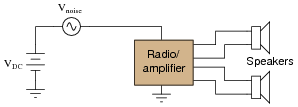What would you suggest as a “fix” for this problem if a friend asked you to apply your electronics expertise to their noisy car audio system? Be sure to provide at least two practical suggestions.

• #### Question 43

A helical resonator is a special type of band-pass filter commonly used in VHF and UHF radio receiver circuitry. Such a device is made up of multiple metal cavities, each containing a helix (coil) of wire connected to the cavity at one end and free at the other. Slots cut between the cavities permits coupling between the coils, with the input at one extreme end and the output at the other: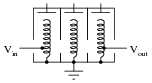The above illustration shows a three-stage helical resonator, with adjustable metal plates at the top of each helix for tuning. Draw a schematic representation of this resonator, and explain where the capacitance comes from that allows each of the coils to form a resonant circuit.

• #### Question 44

 Don’t just sit there! Build something!!

Learning to mathematically analyze circuits requires much study and practice. Typically, students practice by working through lots of sample problems and checking their answers against those provided by the textbook or the instructor. While this is good, there is a much better way.

You will learn much more by actually building and analyzing real circuits, letting your test equipment provide the “answers” instead of a book or another person. For successful circuit-building exercises, follow these steps:

1. Carefully measure and record all component values prior to circuit construction.
2. Draw the schematic diagram for the circuit to be analyzed.
3. Carefully build this circuit on a breadboard or other convenient medium.
4. Check the accuracy of the circuit’s construction, following each wire to each connection point, and verifying these elements one-by-one on the diagram.
5. Mathematically analyze the circuit, solving for all voltage and current values.
6. Carefully measure all voltages and currents, to verify the accuracy of your analysis.
7. If there are any substantial errors (greater than a few percent), carefully check your circuit’s construction against the diagram, then carefully re-calculate the values and re-measure.

For AC circuits where inductive and capacitive reactances (impedances) are a significant element in the calculations, I recommend high quality (high-Q) inductors and capacitors, and powering your circuit with low frequency voltage (power-line frequency works well) to minimize parasitic effects. If you are on a restricted budget, I have found that inexpensive electronic musical keyboards serve well as “function generators” for producing a wide range of audio-frequency AC signals. Be sure to choose a keyboard “voice” that closely mimics a sine wave (the “panflute” voice is typically good), if sinusoidal waveforms are an important assumption in your calculations.

As usual, avoid very high and very low resistor values, to avoid measurement errors caused by meter “loading”. I recommend resistor values between 1 kΩ and 100 kΩ.

One way you can save time and reduce the possibility of error is to begin with a very simple circuit and incrementally add components to increase its complexity after each analysis, rather than building a whole new circuit for each practice problem. Another time-saving technique is to re-use the same components in a variety of different circuit configurations. This way, you won’t have to measure any component’s value more than once.